## Saturday, October 31, 2015

### Relative Mirror Wrapup in DWT: Programmatic Note 6 on Ripples in Mathematics

Introduction

This is my programmatic note 6 on Ch. 4 in "Ripples in Mathematics" by A. Jensen & A. la Cour-Harbo. As I was implementing CDF(2, 2) defined in Ch 3, I was confronted with what Jensen & Cour-Harbo call the boundary problem. The problem shows up when we need to compute the wavelet values at sub-signal boundaries. This is one of those differences between mathematics and computer science. Mathematicians may have the discrete sample time axis stretching from the negative infinity all the way to the positive infinity. But we, the CS folks, must deal with finite signals of some length n that consist of samples enumerated from 0 up to n-1. How are we, for example, supposed to compute a boundary value if the mathematical equation that we are coding up refers to the samples that do not exist.  Say, computing the value at sample 0 may require the values at samples -1 and -2. This is the left boundary problem. Similarly, suppose the length of our signal is n and we need to compute the value at sample n-1 for which the equation requires us to access the samples at positions n and n+1, which do not exist.

One common solution is to return 0's for the samples outside our sub-signal's range. Professor Nievergelt in his excellent book "Wavelets Made Easy" shows that this may not work as well as we would naively expect. Jensen & Cour-Harbo do not seem to explain which method they used in their CDF(2, 2) experiments in Ch. 4. One other method suggested in "Wavelets Made Easy" is mirror reflection. This is what I decided to implement. I may change my implementation in the future as I run experiments with large data sets. But, for now, I will keep experimenting with what I call relative mirror wrapup which I briefly explain below.

Relative Mirror Wrapup

Suppose we have a signal i.e., a 1D array of values: [a(0), a(1), a(2), a(3), a(4), a(5), a(6), a(7)]. When we compute some DWT, especially its ordered version, we must divide the signal into sub-signals and apply the DWT to each sub-signal according to some recurrence. So, we have the start and end of our sub-signal, e.g., s and e, and then we have the discrete sample axis stretching from the negative infinite to the positive infinity. We can align our sub-signal's start (s) with the 0 of the sample axis. Hence, the term relative, because the 0 on the discrete sample axis is positioned relative to the start of the sub-signal. For example,

sample axis:        -3  -2  -1   0       1      2       3      4      5       6      7     8 ....
signal:                              [a(0), a(1), a(2), a(3), a(4), a(5), a(6), a(7)]
sub-signal's start & end:    s       e

The above notation states that our sub-signal is [a(0), a(1)]. Its starting index, s, is 0 and its end index, e, is 1. The notation a(k), 0 <= k <= 7, denotes the individual samples in our signal where k is an array index. The sample axis above the signal is placed so that the start of the sub-signal is aligned with its 0.

Let us define a method that allows us to map the values from the sample axis into the sub-signal indices using the mirror principle. We start with the left boundary. Suppose we need to access a(-1). If we put the mirror at the left boundary of the sub-signal, the reflection of a(-1) is a(0). In other words, the sample axis value of -1 is mapped to the sub-signal's index 0. What about computing a(-3)? The mirror at the left boundary reflects -2 as 1. Similarly, -3 is reflected to 0, and so on.

If we place the mirror at the right boundary, we reflect a(2) to a(1). Note that we cannot simply return a(2), although it really exists in the larger signal, because our sub-signal ends at 1. Consequently, all indices on the sample axis less than 0 and greater than 1 must be reflected back into the sub-signal's indices. Let us consider another example.

sample axis:      -3   -2   -1    0       1       2      3      4       5       6      7     8 ....
signal:                               [a(0), a(1), a(2), a(3), a(4), a(5), a(6), a(7)]
sub-signal's start & end:              s                        e
The beginning of the signal is aligned with the sample axis. The sub-signal starts at 1 and ends at 4. If we place the mirror at the left boundary of the sub-signal, i.e., position 1, then a(0) is reflected to a(1), a(-1) to a(2), a(-2) to a(3), a(-3) to a(4) and then the reflection pattern repeats. If the mirror is placed at the right boundary of the sub-signal, a(5) is reflected to a(4), a(6) to a(3), a(7) to a(2), a(8) to a(1) and then the reflection pattern repeats.

Java Implementation

I have implemented the above logic in the Java class given below.

public class DWTBoundaryHandler {
// relative mirror wrapup
public static int rmw(int s, int e, int i) {
if ( s > e ) throw new IllegalArgumentException("Start = " + s + " > End = " + e);
final int n = (e - s) + 1;
if ( i >= 0 && i < n ) {
return s+i;
}
else {
int r = Math.abs(i) % n;
if ( i < 0 ) {
return ( r != 0 )? s + r -1: e;
}
else {
return ( r != 0 )? e - r: e;
}
}
}

static void run_rmw_test(int s, int e, int is, int ie) { for(int i = is; i <= ie; i++) { display_rmw(s, e, i); }}
// -3  -2  -1    0      1      2       3      4      5       6      7     8 ....
//              [a(0), a(1), a(2), a(3), a(4), a(5), a(6), a(7)]
//                  s      e

public static void rmw_test00() { run_rmw_test(0, 1, -4, -1); run_rmw_test(0, 1,  2,  5); }
// -3  -2  -1    0       1       2      3       4      5      6       7    8 ....
//               [a(0), a(1), a(2), a(3), a(4), a(5), a(6), a(7)]
//                          s=e

public static void rmw_test01(int s) {
display_rmw(s, s, -1);
display_rmw(s, s, -2);
display_rmw(s, s, -3);
display_rmw(s, s, 0);
display_rmw(s, s, 1);
display_rmw(s, s, 2);
display_rmw(s, s, 3);
display_rmw(s, s, 4);
}
//    -3   -2   -1     0      1      2      3       4     5     6     7    8 ....
//                    [a(0), a(1), a(2), a(3), a(4), a(5), a(6), a(7)]
//                                 s                        e

public static void rmw_test02() { run_rmw_test(1, 4, -5, -1); run_rmw_test(1, 4, 4,  8); }

public static void display_rmw(int s, int e, int i) {
System.out.println("rmw(s=" + s + ",e=" + e + ",i=" + i + ")=" + DWTBoundaryHandler.rmw(s, e, i));
}

public static void main(String[] args) { rmw_test02(); }
}

## Monday, October 26, 2015

### Defining Forward DWT through Recurrences: Conceptual Note 1 on DWT Scale Notation in Ripples in Mathematics

Introduction

This is a conceptual note on Ch. 3, pp. 22-23, in "Ripples in Mathematics" by A. Jensen & A. la Cour-Harbo. I had implemented the 1D and 2D Haar Transforms (my github repo is here) before reading this great and beautiful book. So, on my first reading, I quickly read through the notation on p. 22. However, when I started implementing CDF(2, 2), the issue of notation became important. Below are the modifications that I introduced to the DWT scale notation adopted in Ch 3 to understand CDF(2, 2).  The notation for the forward DWT used in Ch. 3 is given in Fig. 1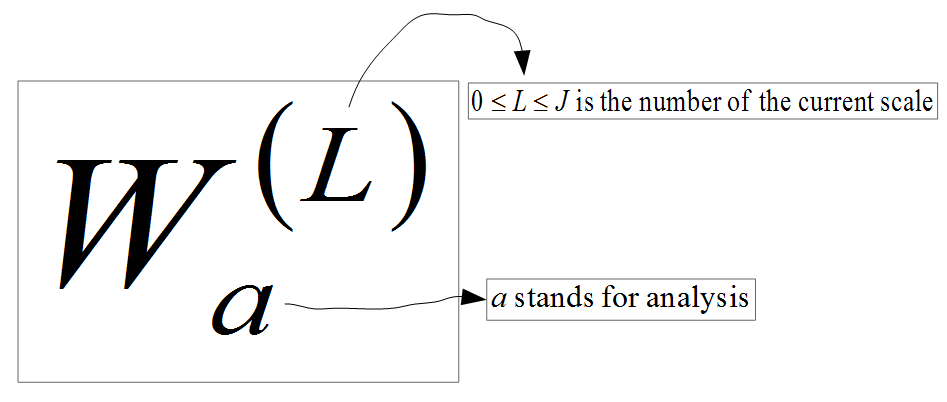Figure 1. Notation for Forward DWT

The forward direct wavelet transform (DWT) is applied to a signal of length 2^J, for J > 0. Each application of the transform is called a scale. The maximum scale value is J.  I take scale 0 to be the original signal. A signal is a sequence of samples, e.g., real numbers. At each scale greater than 0, the signal can be represented as the signal proper, i.e., the scaled signal, and the difference sequence (this term is mine). The difference sequence consists of numbers that allow us to recover the signal at the previous scale and to reason about the signal properties, e.g., how the signal changes from the 1st half to the second half. Fig. 2 shows the notation I used to represent a signal at a specific scale to make sense of the CDF(2, 2) definition on p. 23. This notation differs slightly from the one used in the book and may be helpful to the readers of this document when they implement CDF(2, 2).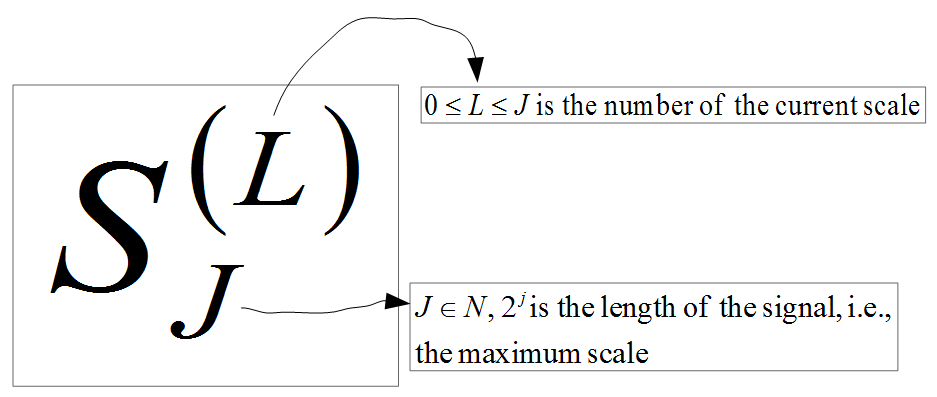Figure 2. Notation for Signal at Scale L
Fig. 3 gives my notation for the i-th sample of a scaled signal. In other words, the symbol defined in Fig. 2 denotes a signal whose individual elements are referenced by symbols defined in Fig. 3.  Note that in Figures 3 and 5, the range for i should be defined as 0 <= i < 2^(J-L), not 0 <= i <= 2^(J-L). I noticed this typo after I published this post.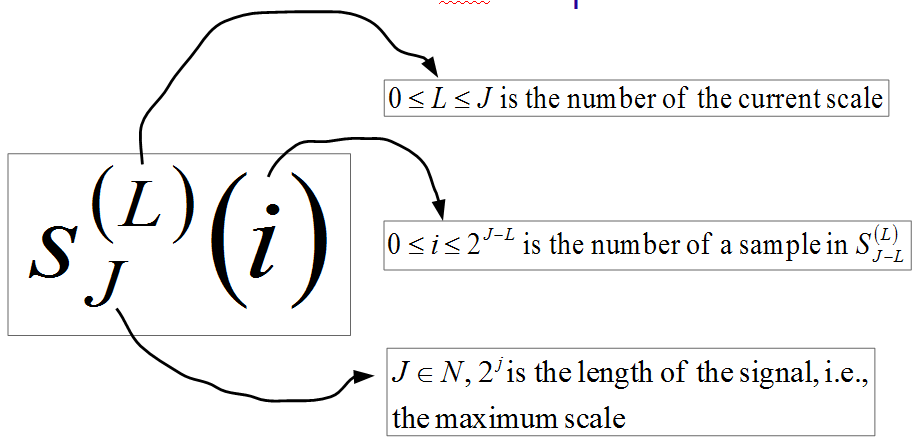Figure 3. Notation for the I-th Sample of the Signal at Scale L
The second component of the DWT at a given scale is the difference sequence. I do not think difference sequence is a standard term. The notation for difference sequences is shown in Fig. 4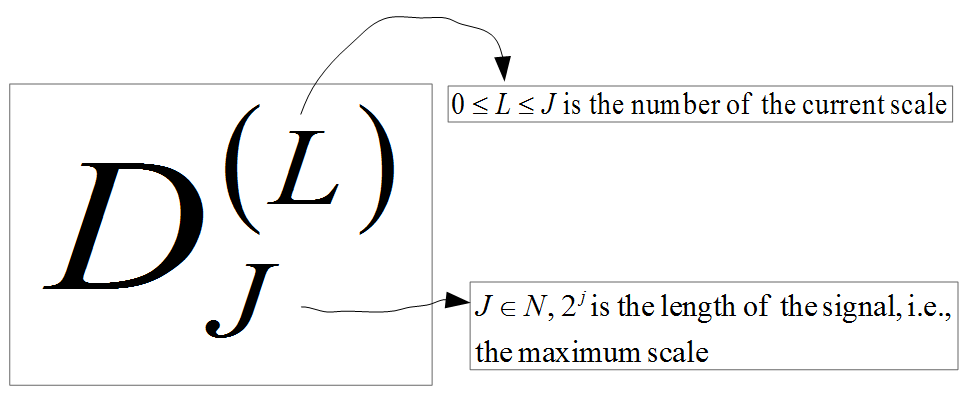Figure 4. Notation for the Difference Sequence at Scale L
Fig. 5 shows the notation used for individual elements of difference sequences.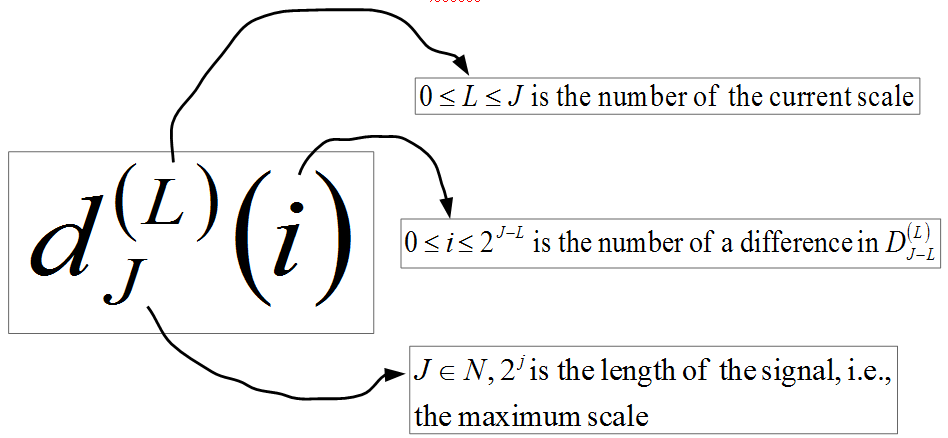Figure 5. Notation for the I-th Difference in the Difference Sequence at Scale L
To denote the DWT of a signal of length 2^J at a scale L, I used the notation given in Fig. 6. T stands for "transform."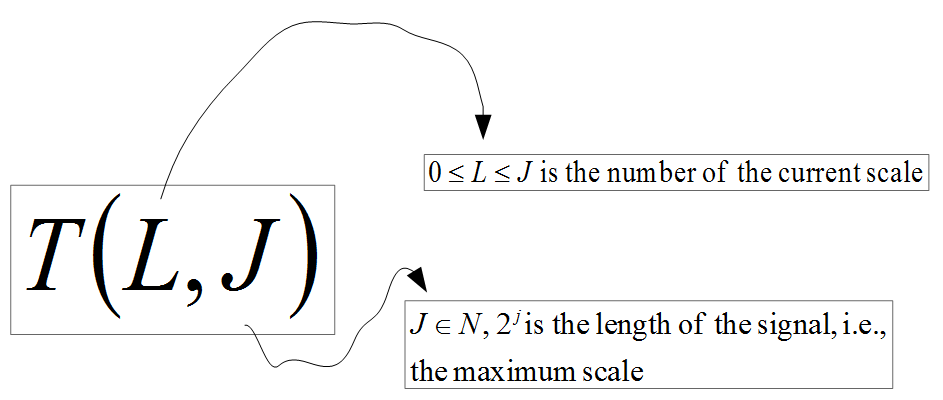Figure 6. Notation for the Direct Wavelet Transform of a Signal of length 2^J at Scale L
Finally, Fig. 7 defines T(L, J) through recurrences.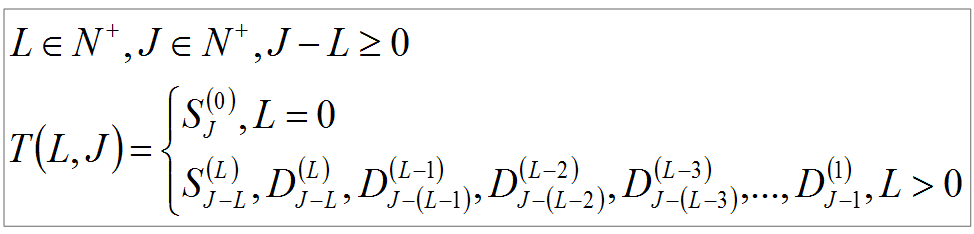Figure 7. Recurrences for T(L, J)

Example 1

Figures 8 - 9 shows the DWT of a signal with 2 samples.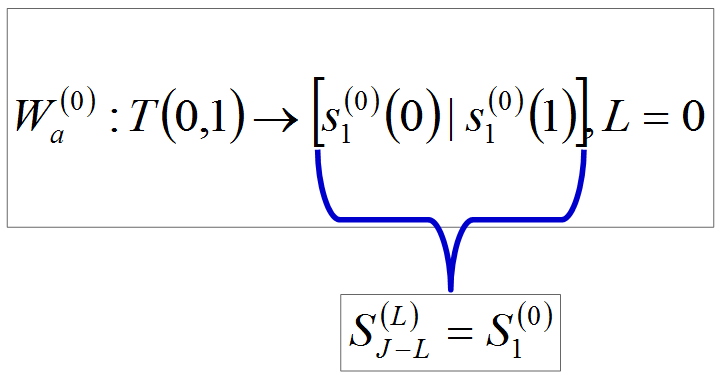Figure 8. T(0, 1)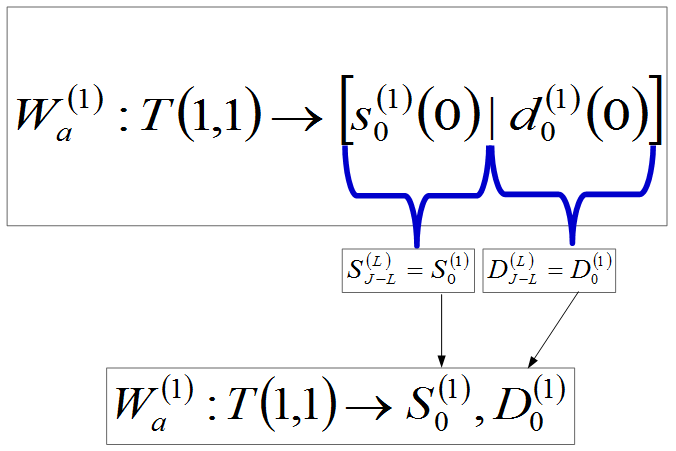Figure 9. T(1, 1)

Example 2

Figures 10 - 12 shows the DWT of a signal with 4 samples.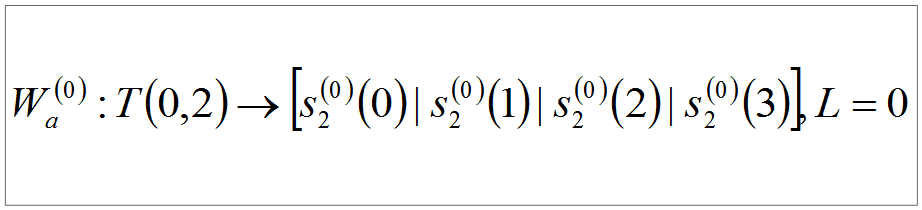Figure 10. T(0, 2)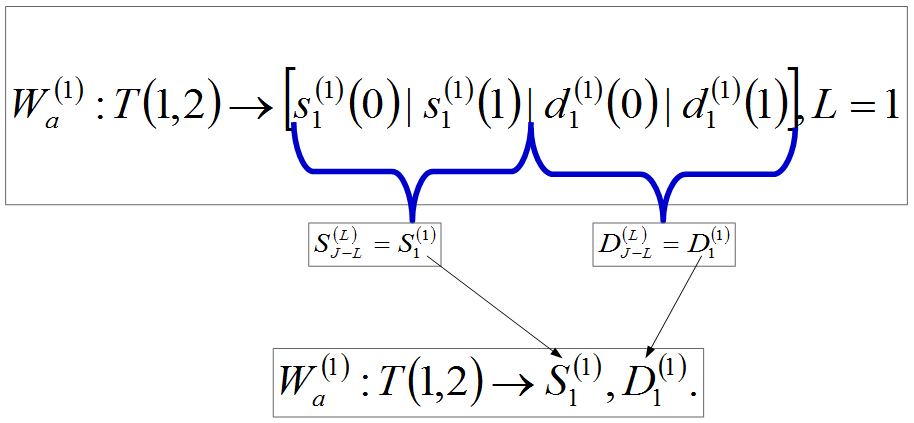Figure 11. T(1, 2)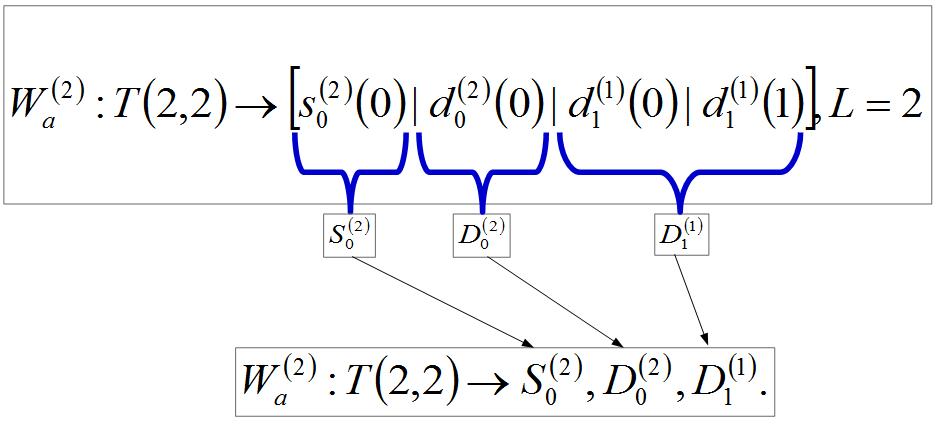Figure 12. T(2, 2)

Example 3

Figures 13 - 16 shows the DWT of a signal with 8 samples.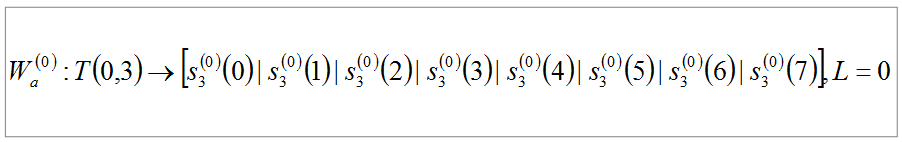Figure 13. T(0, 3)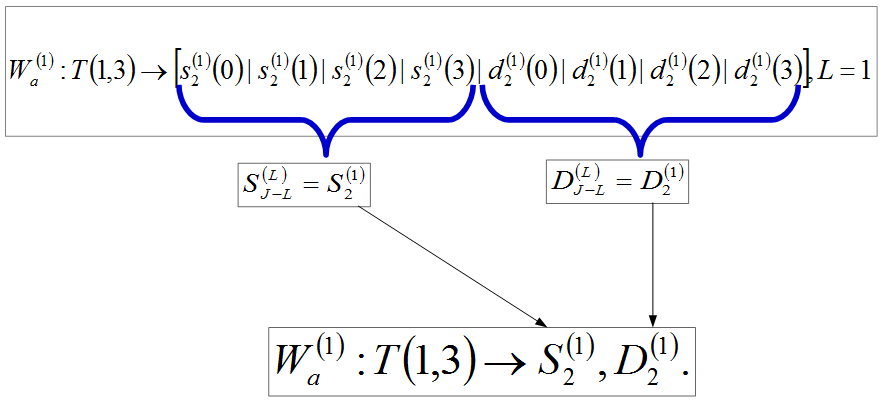Figure 14. T(1, 3)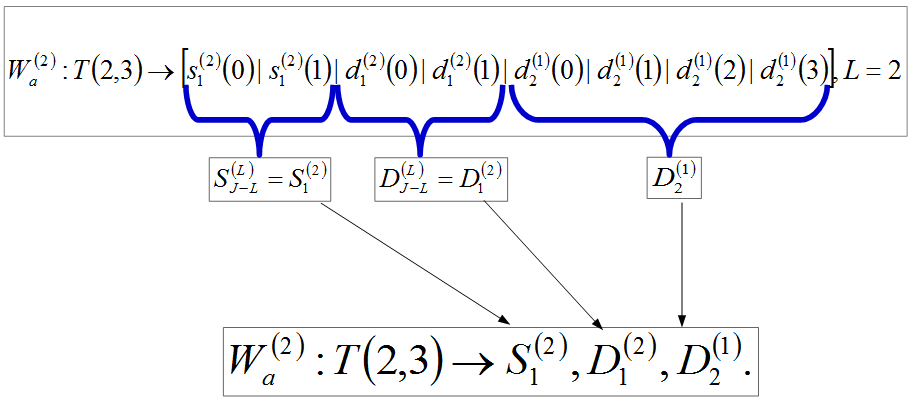Figure 15. T(2, 3)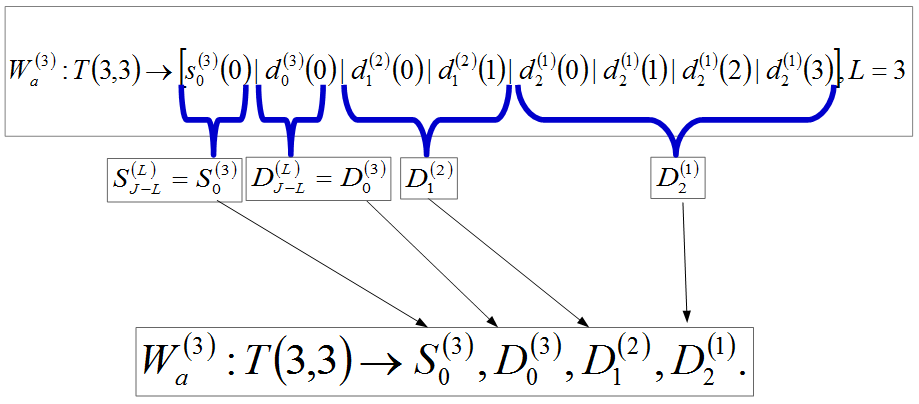Figure 16. T(3, 3)